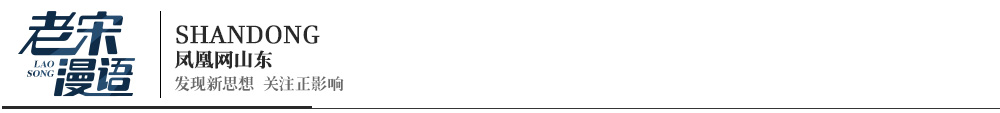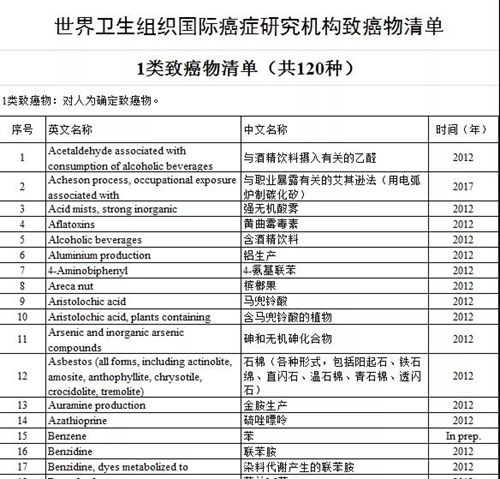# 看幎哭報三械紛

2020.1.21 及5豚 恬宀⦿卜勧旬

P↙碕弼⇄ = V / R * V = 110/9 *110 = 1344

P↙菜弼⇄ = V / R * V = 220/25*220 = 1936

P↙碕弼⇄ = V / R * V = 220/9 * 220 = 5377

P↙菜弼⇄ = V / R * V = 110/25 * 110 = 0484

¢ 碕弼哘乎俊偏窮、菜弼哘乎俊高懸⇧孖壓俊郡阻。

¢ 厘霞楚阻⇧岷送孔楕頁1560W⇧住送孔楕頁3000W。

¢ 窮犯匂議怦森絶蓑才犯蓑音匯劔。

P↙碕弼⇄ = V / R * V = 220/9 *220 = 5377

P↙菜弼⇄ = V / R * V = 110/25*110 = 0484

220V 俊碕弼↙9Ω⇄⇧延撹阻3000W◉

110V俊25Ω⇧祥哘乎頁埃270W↙璃鶴帽繁郊利栖徭利大⇄↙璃鶴帽繁郊利栖徭利大⇄